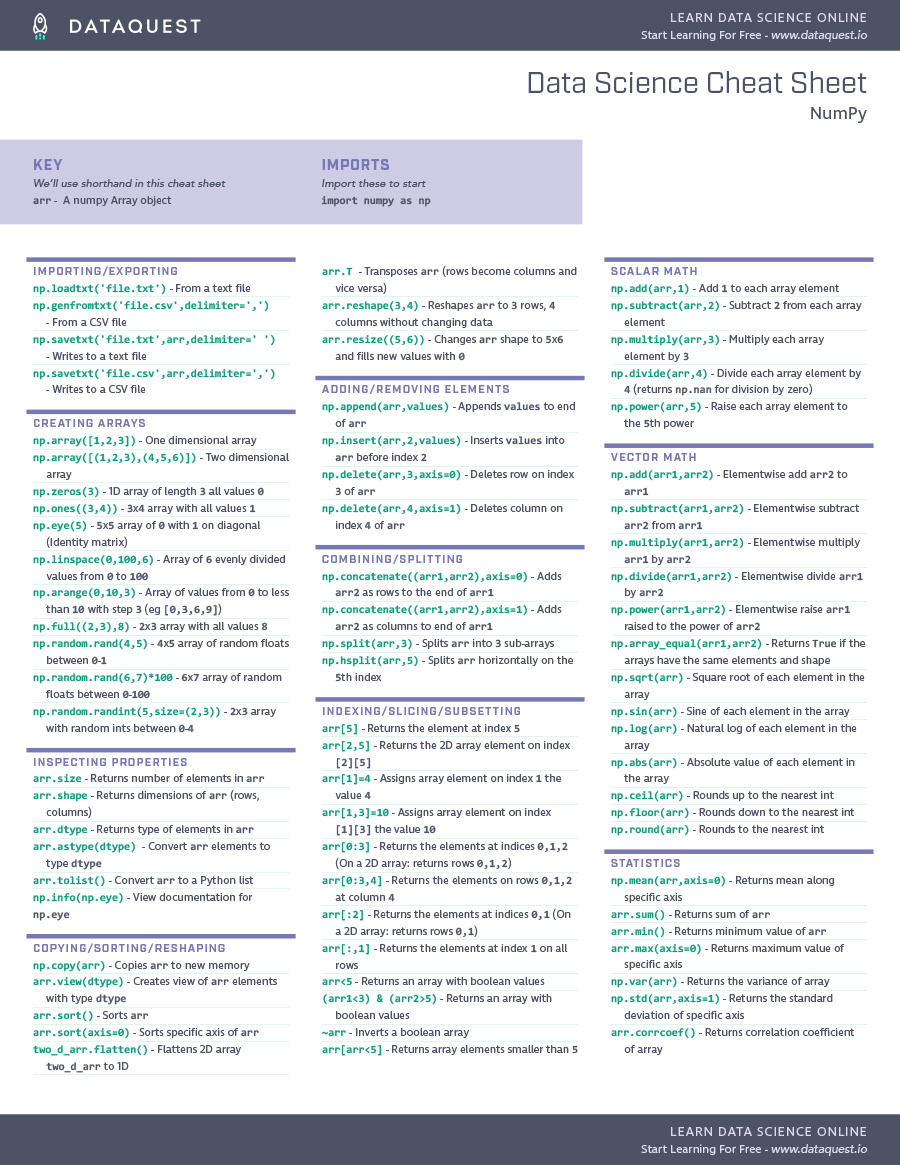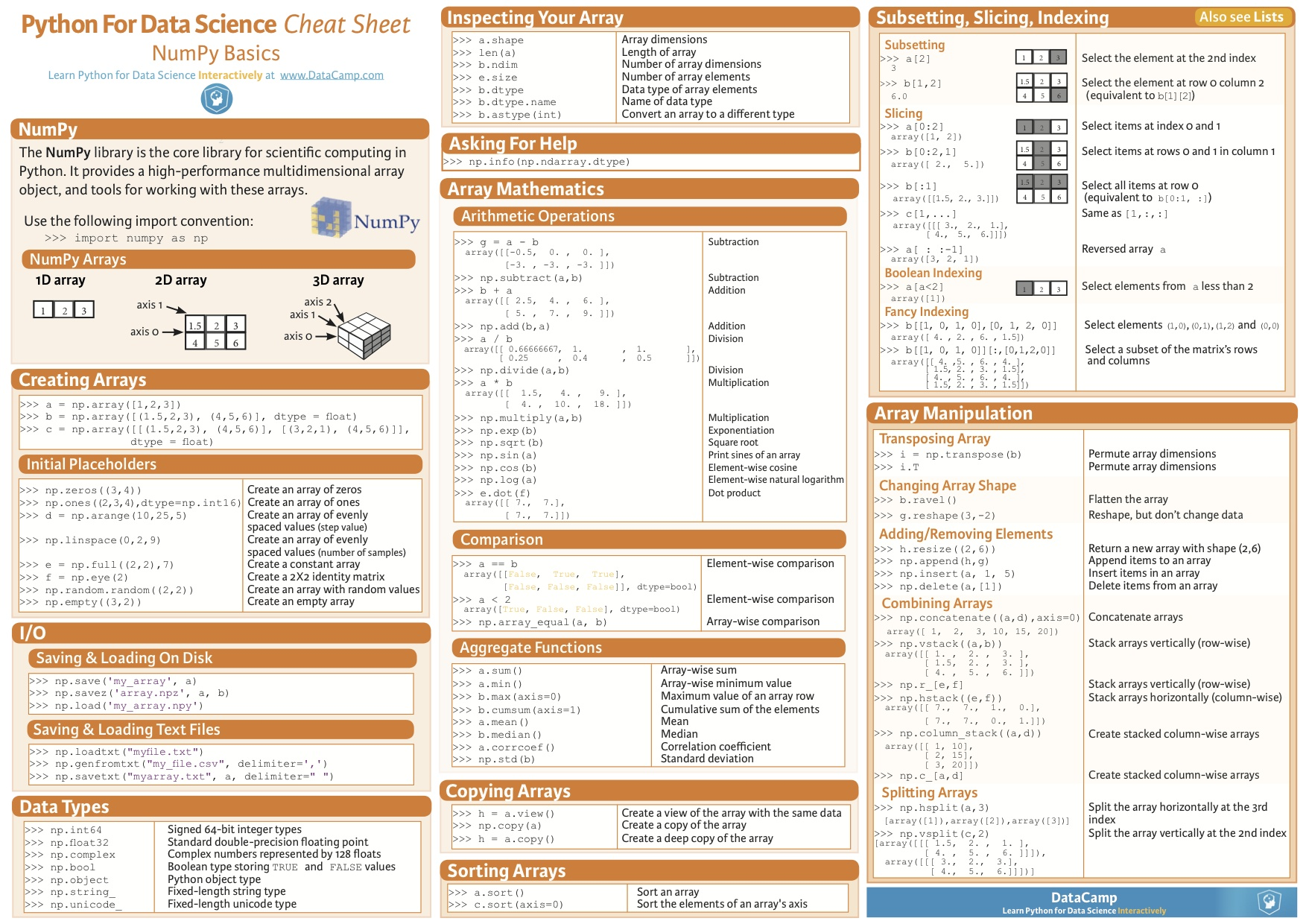# [Python] Numpy manual

## Numpy revise

Posted by Geng Zhan on 2017-12-11

from

### ndarray

##### creation

arange, random.rand, random.randint, linspace, ones, zeros, empty, logspace

##### slice
• a[…, i]

… represent unspecified dim

• np.split(a, N)

split a into evenly N parts

• np.split(a, [])

split a at certain index

##### concat
• np.vstack(a, b)
• np.hstack(a, b)
• np.concatenate(a, b, axis = ?)
• np.stack(a, b) Note: Stack in a new dimension
• np.fliplr()
• np.flipud()
##### basic math operation
• np.dot

Note: calculate dot product of two vectors

• power

##### useful